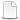HomeTechniques and Tips@RISK for Project 4.xDistribution's Sampling Depends on the Occurrence of an Event

# 12.3. Distribution's Sampling Depends on the Occurrence of an Event

Applies to:
@RISK for Project 4.x
(If you have @RISK 6.0 or newer, use RiskCompound; see Combining Probability and Impact.)

@Risk distributions can also include optional arguments. A useful optional argument is the "EnableWhen( )". Conditions can be specified as the argument for the EnableWhen( ). The condition will determine whether the distribution will be sampled. Example:

Duration = RiskNormal(10,2, EnableWhen(Variable[RiskOccurred]=1) )

The above function will only sample the defined RiskNormal( ) when the global variable called "RiskOccurred" is equal to 1. Conditional statements with EnableWhen( ) can only refer to global variables. However, you can also use the special argument prob=, like this:

Duration = RiskNormal(10,2, EnableWhen(prob=0.5) )

The above function will only sample the defined RiskNormal( ) variable for 50% of the iterations during the simulation. In other words, the RiskNormal( ) has a 50% chance of occurring.

Whenever the EnableWhen( ) evaluates such that the distribution is not sampled, the schedule will be calculated based on the initial value that is in the corresponding field prior to starting the simulation.

Using the EnableWhen( ) function, Risk impact variables can be made dependent on probability-of-occurrence parameters. This is very useful for modeling partially-mitigated and event-based risks.

For example, it is sometimes the case that a mitigation strategy cannot alter the impact of a risk if it occurs. Rather the mitigation strategy will be designed to reduce the likelihood of the risk occurring. Thus, the probability of occurrence for the risk has been reduced. The effect of reducing the probability of occurrence for a risk can be easily modeled using the EnableWhen( ).

See the attached file for an example using the "EnableWhen()" argument.

last edited: 2012-09-11

•EnableWhenExample.mpp